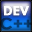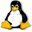Home Latest News Archive Tutorials C++ 2 3 4 Win32 Java OpenGL GLUT OpenGL ES 2 3 4 DirectX VB.NET HTML Photoshop 3dsmax Forums Projects Modelling Links About Us Search Recent Tutorials C / C++ Win32 OpenGL OpenGL ES GLUT## Relational Operators

Tutorials > C++ > Relational Operators

### Introduction

It is almost impossible to make a program worthwhile without allowing it to make certain decisions. To make a decision, your program needs to be able to calculate whether a statement is true or false. This is done using logical and relational operators.

A special data type known as a boolean is a 1 bit data type. It can consist of either a 0 or a 1. The data type is declared in C / C++ by the bool data type.

2 special keywords, true and false. false is equal to 0 and true is equal to 1. We use these keywords usually instead of 0 and 1 as it is easier to read and understand.

Contents of main.cpp :

```#include <stdlib.h>
#include <iostream>

using namespace std;

int main()
{
```

To shorten the code, I have created 2 variables, t and f which have the values of true and false respectively.

```	bool t = true;
bool f = false;
```

You can see that if you output t, you get a 1 and NOT the string "true".

```	cout << t << endl;
```

Our first logical operator that we will discuss is the ! operator. This is known as the NOT operator. It is placed before a variable or expression. It can only be used on a variable or expression that can be represented as true or false. It has the effect of giving the opposite ie.

NOT true is false
NOT false is true

```	cout << "!true : " << !t << endl;
cout << "!false : " << !f << endl;
```

Another operator is the AND (&&) operator. Both expressions need to be true for an && operator to return true. If any of the 2 operands are false, the final answer is false.

```	cout << "true && true : " << (t && t) << endl;
cout << "true && false : " << (t && f) << endl;
cout << "false && false : " << (f && f) << endl;
```

Another operator is the OR (||) operator. Either expression needs to be true for a || operator to return true. If any of the 2 operands are true, the final answer is true.

```	cout << "true || true : " << (t || t) << endl;
cout << "true || false : " << (t || f) << endl;
cout << "false || false : " << (f || f) << endl;
```

Now that you understand how logical operators work, we can mix them with relational operators. Relational operators compare two operands with each other. Some of the relational operators are shown below :

 Operator Description > Greater Than < Less Than >= Greater Than OR Equal To <= Less Than OR Equal To != NOT Equal To == Equal To

Please note that the equal to operator is NOT = but. It must be a double equals (==).

If you said (x == 3), this would return true if x was equal to 3. If you said (x = 3), it would assign 3 to x.

As you may have picked up from the previous statement, the result of any relational comparison is a boolean value.
You may have not seen it before, but you can define more than one variable on the same line if they are the same type as shown below.

```	int x = 3, z = 3;
int y = 6;
```

The statements below give examples of relational expressions. 3 is not greater than 6, therefore (3 > 6) returns false.

```	cout << "3 > 6 : " << (x > y) << endl;
cout << "3 < 6 : " << (x < y) << endl;
cout << "3 == 6 : " << (x == y) << endl;
cout << "3 == 3 : " << (x == z) << endl;

cout << "3 >= 1 : " << (3 >= 1) << endl;

cout << "3 != 1 : " << (3 != 1) << endl;
```

Relational expressions returning boolean values allow us to mix them with logical expressions.
The first statement in the next code segment will evaluate as follows :

1.     ((3 < 9) || (7 > 2))
2.     ( true || true )
3.     ( true )
```	cout << "3 < 9 OR 7 > 2 : " <<
((3 < 9) || (7 > 2)) << endl;
```

Similarly, the next statement will evaluate as follows :

1.     ((4 != 6) && (10 > 12))
2.     ( true || false )
3.     ( false )
```	cout << "4 != 6 AND 10 > 12 : " <<
((4 != 6) && (10 > 12)) << endl;

system("pause");

return 0;
}```

Congratulations. You should now know how to create logical and relational expressions using special operators. The operators will become second nature to you as they are the basis of every program. You may be wondering how these expressions can help you? You will be able to see this more clearly in the next tutorial.

Please let me know of any comments you may have : Contact Me

 Source Files :< Tutorial 11 - Arithmetic Operators Tutorial 13 - If Statement >